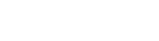# Strong Homomorphic Encryption

## Introduction:

Encryption programs are genuinely designed to destroy the relation between plain text and the corresponding ciphertext. A good encryption algorithm produces a cipher text that can not be distinguished from a random number. The only way to know the actual message is by having a decryption algorithm.

Such decryption can only be done when mathematical operation can be performed on ciphertext which helps to yield the plain text. Homomorphic Encryptions have achieved these mutual goals of strong encryption and ability of performing strong algorithms. Let us discuss Homomorphic Encryption.

## Homomorphic Encryption:

Homomorphic encryption has provided programmers with a strong encryption algorithm with a facility to apply arithmetic operations on the encrypted text. While there are multiple ways to achieve homomorphic encryption we will discuss a few techniques in our publication today.

So let us discuss a general idea of this method. We suppose a natural number of natural numbers, for instance 6 now if we have to encrypt a number suppose 2 we will make 2 the exponential value of 6 for which we will get.

62=36

Where 36 is the encrypted value for 2. Now if we want to multiply the encrypted value by 3 we can easily do it by increasing the exponential value as shown below.

(62)3=62.3=66=46,656

Similarly in the same way we can perform addition and subtraction in order to encrypt our number 2.  However since the base 6 is public we can easily decrypt it by dividing it by number 5 until we get 1 as the answer. Hence the number of steps is the secret key to the encryption.

## Modular Arithmetic:

The modular arithmetic plays an important role in performing the arithmetic operation during the encryption. We suppose first n natural numbers if any number that is outside the range that we have defined then we wrap that number according to our range. For instance let us choose the first 8 numbers of the natural number and we represent it in a circle with equal 8 ticks.

Now our next step will be to have a rope of length 10 units. We wrap it around the circle. After one rotation we will have the rope left so we will continue the process and it will end at number 2. Hence the example illustrates the modulo function that no matter what is the length of the rope it will always end on the number that lies within the range. So does the negative number the only difference is when we have a negative number we start our rotation anticlockwise. So let us illustrate another example where we use the function mod n. We suppose our range upto first 6 numbers which can be written as below.

3*5=3 (mod 6)

5+2=1 (mod 6)

Most importantly the order of the operation does not matter. We can first perform all the operations and then apply the modulo function or vice versa.

So now you must be wondering why modulo function is so important for our encryption. The reason behind its importance is that it provides us with the non trivial solution. And many combinations can have the same solution.

## Strong Homomorphic Encryption:

If we go back to our previous understanding of homomorphic encryption and apply modular arithmetic with modulo 7 we get the following results

51=5(mod 7)

52=4(mod 7)

53=6(mod 7)

And different exponents will have the same results

55=3(mod 7)

511=3(mod 7)

517=3(mod 7)

This makes it hard for the hacker to find the exponent. And if the modulo is large enough it becomes impossible to find the exponents and most of the modern day cryptography is based on the hardness of the problem.

## Conclusion:

The homomorphic encryption has been very interesting but sadly it never got its due attention. With the changing time and modern encryption have realised its importance and have been used in ZK-SNARK which will be discussed in our next publication.## Get notified on our latest Web3 researches and catch Xord at a glance.

By checking this box , I agree to receive email communication from Xord.We research blockchain core and infrastructure while developing products for the Web3 ecosystem in addition to providing blockchain development services, audit & tokenomics, along with design in the Web3 space.

Explore
Write-Ups
Services